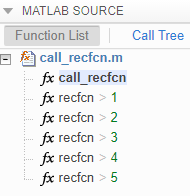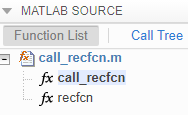## Force Code Generator to Use Run-Time Recursion

When your MATLAB® code includes recursive function calls, the code generator uses compile-time or run-time recursion. With compile-time recursion, the code generator creates multiple versions of the recursive function in the generated code. These versions are known as function specializations. With run-time recursion, the code generator produces a recursive function. If compile-time recursion results in too many function specializations or if you prefer run-time recursion, you can try to force the code generator to use run-time recursion. Try one of these approaches:

### Treat the Input to the Recursive Function as a Nonconstant

Consider this function:

```function y = call_recfcn(n) A = ones(1,n); x = 5; y = recfcn(A,x); end function y = recfcn(A,x) if size(A,2) == 1 || x == 1 y = A(1); else y = A(1)+recfcn(A(2:end),x-1); end end ```

`call_recfcn` calls `recfcn` with the value 5 for the second argument. `recfcn` calls itself recursively until `x` is 1. For each `recfcn` call, the input argument `x` has a different value. The code generator produces five specializations of `recfcn`, one for each call. You can see the specializations in the MATLAB Function report.To force run-time recursion, in `call_recfcn`, in the call to `recfcn`, instruct the code generator to treat the value of the input argument `x` as a nonconstant value by using `coder.ignoreConst`.

```function y = call_recfcn(n) A = ones(1,n); x = coder.ignoreConst(5); y = recfcn(A,x); end function y = recfcn(A,x) if size(A,2) == 1 || x == 1 y = A(1); else y = A(1)+recfcn(A(2:end),x-1); end end ```

In the MATLAB Function report, you see only one specialization.### Make the Input to the Recursive Function Variable-Size

Consider this code:

```function z = call_mysum(A) %#codegen z = mysum(A); end function y = mysum(A) coder.inline('never'); if size(A,2) == 1 y = A(1); else y = A(1)+ mysum(A(2:end)); end end```

If the input to `mysum` is fixed-size, the code generator uses compile-time recursion. To force the code generator to use run-time conversion, make the input to `mysum` variable-size by using `coder.varsize`.

```function z = call_mysum(A) %#codegen B = A; coder.varsize('B'); z = mysum(B); end function y = mysum(A) coder.inline('never'); if size(A,2) == 1 y = A(1); else y = A(1)+ mysum(A(2:end)); end end```

### Assign Output Variable Before the Recursive Call

The code generator uses compile-time recursion for this code:

```function y = callrecursive(n) x = 10; y = myrecursive(x,n); end function y = myrecursive(x,n) coder.inline('never') if x > 1 y = n + myrecursive(x-1,n-1); else y = n; end end```

To force the code generator to use run-time recursion, modify `myrecursive` so that the output `y` is assigned before the recursive call. Place the assignment `y = n` in the `if` block and the recursive call in the `else` block.

```function y = callrecursive(n) x = 10; y = myrecursive(x,n); end function y = myrecursive(x,n) coder.inline('never') if x == 1 y = n; else y = n + myrecursive(x-1,n-1); end end```